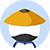Tanya prepared 4 different letters to be sent to 4 different addresses. For each letter, she prepared an envelope with its correct address. If the 4 letters are to be put into the 4 envelopes at random, what is the probability that only 1 letter will be put into the envelope with its correct address?
• A$\frac{1}{24}$
• B$\frac{1}{8}$
• C$\frac{1}{4}$
• D$\frac{1}{3}$
• E3/8

• 网友解析

### 题目讨论

• 优质讨论
• 最新讨论• 所属科目:数学PS
• 题目来源1:PREP07 Test 2-1
• 正确率: 37%
• 难度: